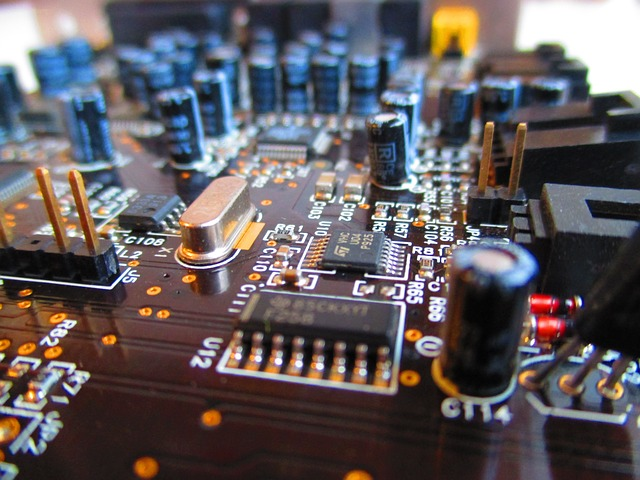# DC CircuitsDC circuits are the basic building blocks of computers. By the end of this Module, you’ll be able to recognize and understand some of the components on this circuit board.

Welcome to Module 3, our introductory guide to DC circuits. Now that we have a good grasp of electrical theory, we’ll build on the concepts introduced in Modules 1 and 2 to introduce you, step by step, to the components and functionality of DC circuits. We’ll be covering the basics of Direct Current circuits including resistors in series and parallel, and capacitors. Along the way we’ll deepen our knowledge about important topics like voltage, current, and resistance. Finally, we’ll be using this knowledge to develop a comprehensive understanding of more complex circuits including voltage dividers and RC circuits.

DC Circuits are incredibly important. Most electronics use DC circuits including the computer, tablet, or phone that you are probably reading this website on. It’s also the best way to start learning about electrical theory. You’ll quickly learn almost all of the important concepts. You can explore many of the important electronic devices (such as resistors, capacitors, LEDs and lasers). Some of these will be covered in the modules on DC circuits, and others will be discussed in our modules on semiconductors.

Once you have a solid understanding of DC circuits, you can skip the section on AC circuits if you’d like. However, it is helpful to understand some AC devices like transformers so we’d recommend at least skimming that material.

On with the show! Let’s learn about Direct Current Circuits in Lesson 1: Introduction to DC Circuits.

Module 3 – DC Circuits

Lesson 0: Introduction to Module 3

Lesson 1: Introduction to DC Circuits

Lesson 2: Series and Parallel Circuits

Lesson 3: DC Power Sources and Batteries

Lesson 4: Resistors, Capacitors, and Inductors

Lesson 5: Resistors in Series

Lesson 6: Resistors in Parallel

Lesson 7: Voltage Dividers

Lesson 8: Kirchoff’s Current Law (KCL)

Lesson 9: Kirchoff’s Voltage Law (KVL)

Lesson 10: Capacitors

Lesson 11: Dielectric Materials

Lesson 12: Capacitors in Parallel

Lesson 13: Capacitors in Series

Lesson 14: Capacitors in Series and Parallel